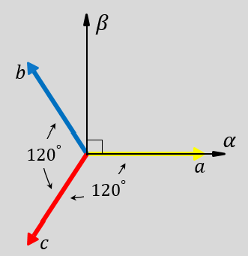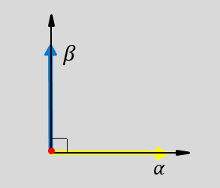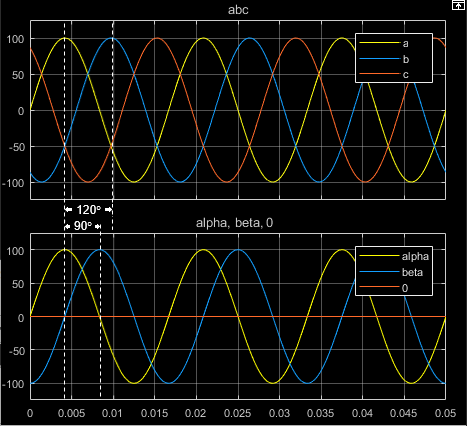# Clarke Transform

Implement abc to αβ0 transform

•Libraries:
Simscape / Electrical / Control / Mathematical Transforms

## Description

The Clarke Transform block converts the time-domain components of a three-phase system in an abc reference frame to components in a stationary ɑβ0 reference frame. The block can preserve the active and reactive powers with the powers of the system in the abc reference frame by implementing a power invariant version of the Clarke transform. For a balanced system, the zero component is equal to zero.

The figures show:

• The direction of the magnetic axes of the stator windings in the abc reference frame and the stationary ɑβ0 reference frame• Equivalent ɑ, β, and zero components in the stationary reference frame• The time-response of the individual components of equivalent balanced abc and ɑβ0 systems### Equations

The block implements the Clarke transform as

`$\left[\begin{array}{c}\alpha \\ \beta \\ 0\end{array}\right]=\frac{2}{3}\left[\begin{array}{ccc}1& -\frac{1}{2}& -\frac{1}{2}\\ 0& \frac{\sqrt{3}}{2}& -\frac{\sqrt{3}}{2}\\ \frac{1}{2}& \frac{1}{2}& \frac{1}{2}\end{array}\right]\left[\begin{array}{c}a\\ b\\ c\end{array}\right],$`

where:

• a, b, and c are the components of the three-phase system in the abc reference frame.

• α and β are the components of the two-axis system in the stationary reference frame.

• 0 is the zero component of the two-axis system in the stationary reference frame.

The block implements the power invariant version of the Clarke transform as

`$\left[\begin{array}{c}\alpha \\ \beta \\ 0\end{array}\right]=\sqrt{\frac{2}{3}}\left[\begin{array}{ccc}1& -\frac{1}{2}& -\frac{1}{2}\\ 0& \frac{\sqrt{3}}{2}& -\frac{\sqrt{3}}{2}\\ \sqrt{\frac{1}{2}}& \sqrt{\frac{1}{2}}& \sqrt{\frac{1}{2}}\end{array}\right]\left[\begin{array}{c}a\\ b\\ c\end{array}\right].$`

## Ports

### Input

expand all

Components of the three-phase system in the abc reference frame.

Data Types: `single` | `double`

### Output

expand all

Alpha-axis component,α, beta-axis component β, and zero component in the stationary reference frame.

Data Types: `single` | `double`

## Parameters

expand all

Preserve the active and reactive power of the system in the abc reference frame.

 Krause, P., O. Wasynczuk, S. D. Sudhoff, and S. Pekarek. Analysis of Electric Machinery and Drive Systems. Piscatawy, NJ: Wiley-IEEE Press, 2013.